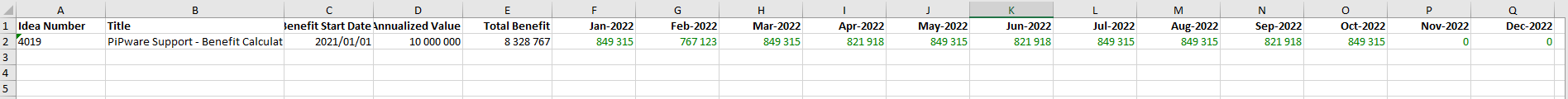The Actual Benefit needs to be the same between the Benefits and the Cumulative Benefits.
• NB: The Annualized Benefit filter option determines which benefit value (Annualized Benefit or Benefit For Month) is displayed on the Cumulative Benefits report.

The calculation is explained below:

• Note this Idea has an Idea Value of 10 000 000 (displayed below as the Target Annual Benefit).The Benefit summary section is calculated using the following values i.e  Benefit for Jan 2022.
• Idea Value(10 000 000) obtained from the VDT.
• Month days (31).
• Total Benefit days (365)

See this for more details: Idea Benefit - New Grid

Using the values above the Summary is Calculated as follows:

• Benefit for Month of Jan 2022 = (Idea Value/benefit days * MonthDays) = ((10M / 365)* 23) = 849 315,07.
• Target Monthly Benefit for Jan 2022 = (Idea Value/benefit days *MonthDays ) = ((10M / 365)* 23) = 849 315,07.
• Annualized Benefit for Jan 2022 = (Annualized benefit from the Actual's) = 10 M
• Target Annual Benefit = Idea Value = 10 M
• % of Target Improvement Achieved = (Annualized Benefit / Idea Value) = ((10 M/ 10 M) * 100) = 100%.
• Variance vs Target Annual Benefit = (Annualized Benefit- Idea Value) = (10 M - 10 M) = 0.

The cumulative Benefits (\$) Report download will have values as displayed on the Idea Benefits (\$) Summary.The Details section of the Cumulative Benefits report is calculated as follows:

This is used to compare the achieved Benefit with the Target that was supposed to be delivered for the particular month and variances.E.,g.
• Target Value that was meant to be achieved for the month of Jan-2022 ( 849 315,07).
• Actual benefit value that was achieved for the month Jan-2022 (849 315,07 ).
• Variance = (Actual - Target) = ( 849 315,07  - 849 315,07 ) = 0.
• Variance % = (849 315,07  - 849 315,07 ) * 100 = 0 %